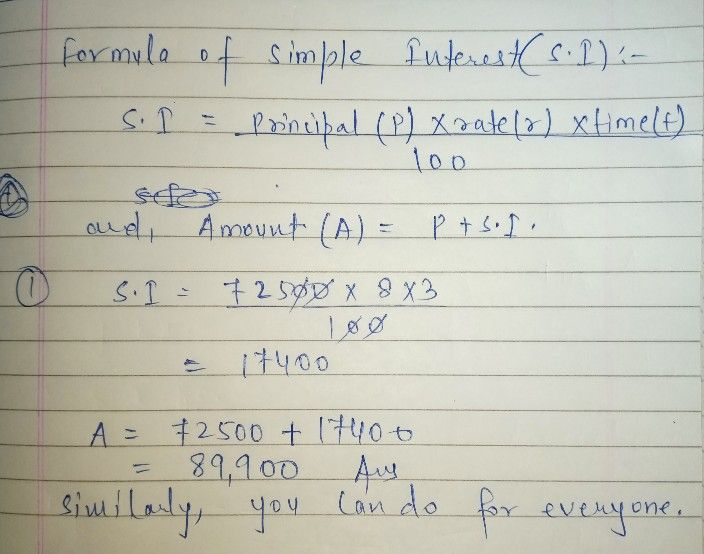Symbol
Problem. 1. $P2000000$ 2. $P24a000$ 3. 4% $2na$ $\left(4cc$ $aa∠-\left(2e\left(cc4c$ $s=$ Complete the table by finding the simple interest. Principal (P) Interest Rate (r) $im0$ (t) $amoan$ of Interest ) 1. $P72500$ 8% $3yr$ 2. $P3000$ 12% $6n10$ 32 $P48200$ 11% $2\dfrac {1} {2}y$ 4. $P161500$ $10\dfrac {4} {5}$ $4yr$ 5. $P450000$ $9\dfrac {1} {4}$ $5y\pi$ Complete the table by finding the maturity value. Principal (P) Interest Rate (r) Time (t) Maturity Value (A 6. $P35600$ 6% 9 mo
10th-13th grade
Other
Search count: 132
SolutionQanda teacher - aftabalamplease provide some gift coins and also please evaluate and like ?
if you are satisfy with my answer